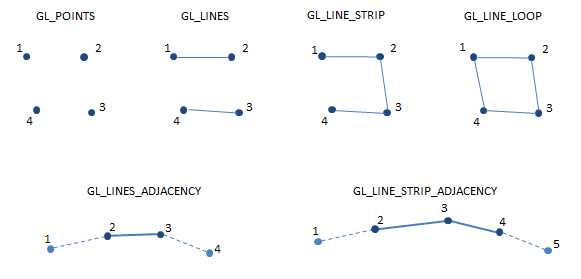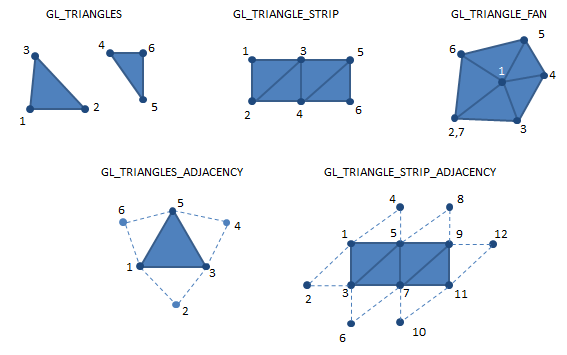The primitive assembly stage receives as input the processed vertices from the vertex shader, and the vertex connectivity information from the application as specified by in the `glDraw*` family of OpenGL commands.

The vertex connectivity states how the vertices are connected to create a primitive. Primitives can be points, lines, triangles, or patches. Furthermore, adjacency information can also be made available, i.e. the application may also provide the vertices that make up the adjacent primitives. This information is of use only  for the geometry shader, and, if not such shader is active, the adjacency information will be ignored.

As output the primitive assembly produces primitives or patches. For instance, if the input is a sequence of six vertices, and the connectivity information specifies that we are using triangles, the output will be two triangles. For triangle strips, and considering also six vertices, the output will be four triangles.

Patches are the primitive types accepted by the tessellation control shader, available in OpenGL 4+. The number of vertices of  patch is not fixed as in the primitives case, and it can vary between 1 and an implementation dependent constant, `GL_MAX_PATCH_VERTICES`, vertices per patch.

Graphically, assuming for instance that the primitive type is GL_TRIANGLES, this can be depicted as follows:The following table shows the possible input connectivity information settings, and the outputs produced, as well as the shaders that use them.

 glDraw command output primtive used in shaders `GL_POINTS` `points` geometry; fragment `GL_LINES` `lines` geometry; fragment `GL_LINE_STRIP` `lines` geometry; fragment `GL_LINE_LOOP` `lines` geometry; fragment `GL_LINES_ADJACENCY` `lines_adjacency` geometry `GL_LINE_STRIP_ADJACENCY` `lines_adjacency` geometry `GL_TRIANGLES` `triangles` geometry; fragment `GL_TRIANGLE_STRIP` `triangles` geometry; fragment `GL_TRIANGLE_FAN` `triangles` geometry; fragment `GL_TRIANGLES_ADJACENCY` `triangles_adjacency` geometry `GL_TRIANGLE_STRIP_ADJACENCY` `triangles_adjacency` geometry `GL_PATCHES` `patches` tessellation control

Bellow are examples of what can be obtained with points, lines, and triangles. Regarding primitives with adjacency information, the full lines indicate the main primitive, and the dashed lines connect the adjacent vertices. Note the ordering in the graphics, all primitives are ordered counter clock wise.The geometrical interpretation of a patch is not as linear, so it is not represented in here. See the section on tessellation for more info on patches.